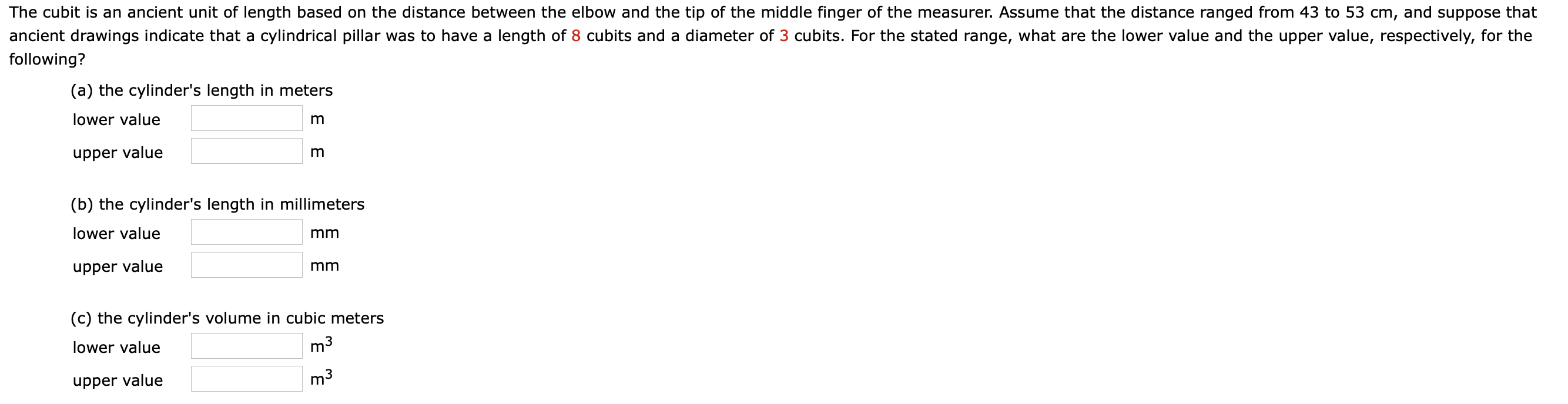# The cubit is an ancient unit of length based on the distance between the elbow and the tip of the middle finger of the measurer. Assume that the distance ranged from 43 to 53 cm, and suppose thatancient drawings indicate that a cylindrical pillar was to have a length of 8 cubits and a diameter of 3 cubits. For the stated range, what are the lower value and the upper value, respectively, for thefollowing?(a) the cylinder's length in meterslower valueupper value(b) the cylinder's length in millimeterslower valuemmupper valuemm(c) the cylinder's volume in cubic meterslower valuem3upper valuem3

Question
36 views

Help with ahelp_outlineImage TranscriptioncloseThe cubit is an ancient unit of length based on the distance between the elbow and the tip of the middle finger of the measurer. Assume that the distance ranged from 43 to 53 cm, and suppose that ancient drawings indicate that a cylindrical pillar was to have a length of 8 cubits and a diameter of 3 cubits. For the stated range, what are the lower value and the upper value, respectively, for the following? (a) the cylinder's length in meters lower value upper value (b) the cylinder's length in millimeters lower value mm upper value mm (c) the cylinder's volume in cubic meters lower value m3 upper value m3 fullscreen
check_circle

Step 1

(a)

Convert the lower and upper value of a cubit into meters and then multiply by 8 since the length the cylinder is 8 cubits.

Step 2

### Want to see the full answer?

See Solution

#### Want to see this answer and more?

Solutions are written by subject experts who are available 24/7. Questions are typically answered within 1 hour.*

See Solution
*Response times may vary by subject and question.
Tagged in

### Other